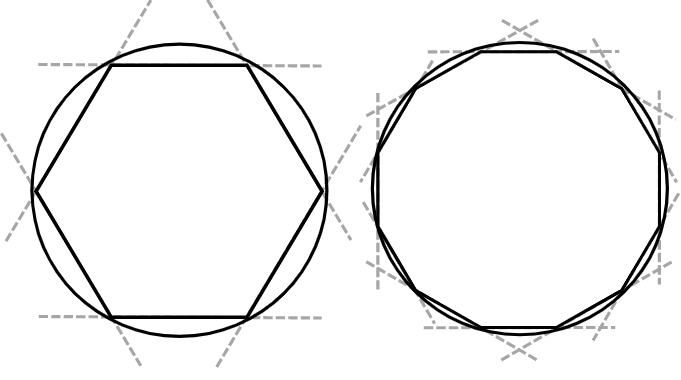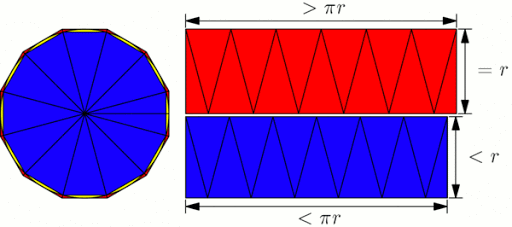## ArchimedesArchimedes of Syracuse was a Greek mathematician, physicist, engineer, inventor, and astronomer. He is regarded as one of the leading scientists in classical antiquity. Archimedes anticipated modern calculus and analysis by applying concepts of infinitesimals and the method of exhaustion to derive and prove a range of geometrical theorems.

Estimating πIn his treatise Measurement of a Circle, he did this by drawing a larger regular hexagon outside a circle and a smaller regular hexagon inside the circle. The number of sides of each regular polygon were progressively doubled and the side length of each polygon calculated at every step. As the number of sides increased, the polygons became a more accurate approximation of a circle. After four such steps, when the polygons had 96 sides each, he was able to determine that the value of π lay between 3 1/7 (approx. 3.1429) and 3 10/71 (approx. 3.1408), consistent with its actual value of approximately 3.1416.

Area of the CircleHe also proved that the area of a circle was equal to π multiplied by the square of the radius of the circle (πr² ). In his treatise “On the Sphere and Cylinder”, Archimedes postulated that any magnitude when added to itself enough times will exceed any given magnitude. This is the Archimedean property of real numbers